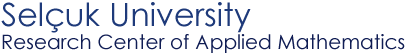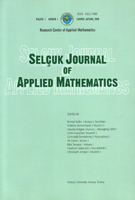Selçuk Journal of Applied Mathematics www.selcuk.edu.trSelçuk Journal of
Applied Mathematics

Winter-Spring, 2003
Volume  4
Number 1

Research Center of
Applied Mathematics

 SJAM Winter-Spring 2003, Volume 4 - Number 1 On location of the matrix spectrum inside an elipse* Ayţe Bulgak1 , G. Demidenko2, and I. Matveeva2* 1 Research Centre of Applied Mathematics,   Selçuk University, Konya, Turkey;   Email : abulgak@selcuk.edu.tr 2 Sobolev Institute of Mathematics,   SB RAS, 630090 Novosibirsk, Russia;   Email: demidenk@math.nsc.ru,   Email: matveeva@math.nsc.ru Received: July 3, 2002 Summary In the present article we consider the problem on location of the spectrum of an arbitrary matrix $A$ inside the ellipse $${\cal E} = \{\lambda \in C: \ \frac{(\Re\lambda)^2}{a^2} + \frac{(\Im\lambda)^2}{b^2} = 1\}, \quad a > b.$$ One of the authors (see~) established a connection of the problem with solvability of the matrix equation $$H - \left(\frac{1}{2a^2} + \frac{1}{2b^2}\right)A^*HA - \left(\frac{1}{4a^2} - \frac{1}{4b^2}\right) (HA^2 + (A^*)^2H) = C.$$ In this article we construct a Hermitian positive definite solution $H$ to the equation in the form of a power series. We prove that the norm $\|H\|$ characterizes an immersion depth of eigenvalues of the matrix $A$ in the inside of the ellipse ${\cal E}$. On the base of these results we propose an algorithm to determine whether the spectrum of the matrix $A$ belongs to the inside of the ellipse ${\cal E}$. Key words ellipse, matrix spectrum, matrix equations, matrix series, algorithm Mathematics Subject Classification (2000): 15A18, 15A24, 65F30 (*) The research was financially supported by the Scientific and Technical Research Council of Turkey (TUBITAK) in the framework of the NATO-PC Fellowships Programme. Article in PS format (417 kb) Article in ZIP format (197 kb)
 The article is available to read in  Ghostview format
 [Home] [contact]